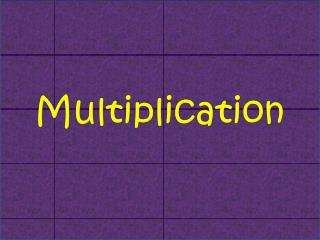Download PresentationMultiplication# Multiplication - PowerPoint PPT Presentation

Download Presentation##### Multiplication

Download Policy: Content on the Website is provided to you AS IS for your information and personal use and may not be sold / licensed / shared on other websites without getting consent from its author. While downloading, if for some reason you are not able to download a presentation, the publisher may have deleted the file from their server.

- - - - - - - - - - - - - - - - - - - - - - - - - - - E N D - - - - - - - - - - - - - - - - - - - - - - - - - - -
##### Presentation Transcript

1. Multiplication

2. multiply times X lotsof

3. Here are some arrays to show what multiply means. 3 x 2 = 6 3 lots of 2 are 6 5 x 2 = 10 5 lots of 2 are 10

4. When we multiply, we are really adding together lots of the same number. + 2 + 2 + 2 = 8 Four lots of 2 are 8 4 x 2 = 8

5. 1 lot of 2 is 2 1 x 2 = 2 2 lots of 2 are 4 2 x 2 = 4 3 lots of 2 are 6 3 x 2 = 6 4 lots of 2 are 8 4 x 2 = 8 5 lots of 2 are 10 5 x 2 = 10 6 lots of 2 are 12 6 x 2 = 12 7 lots of 2 are 14 7 x 2 = 14 8 lots of 2 are 16 8 x 2 = 16 9 lots of 2 are 18 9 x 2 = 18 10 lots of 2 are 20 10 x 2 =20

6. So, let’s look at what the 2 times table means: 1 x 2 = 2 2 + 0 = 2 (1 lot of 2) 2 x 2 = 4 2 + 2 = 4 (2 lots of 2) 3 x 2 = 6 2 + 2 + 2 = 6 (3 lots of 2) 4 x 2 = 8 2 + 2 + 2 + 2 = 8 (4 lots of 2) and so on. 5 x 2 = 10 2 + 2 + 2 + 2 + 2 = 10 6 x 2 = 12 2 + 2 + 2 + 2 + 2 + 2 = 12 7 x 2 = 14 2 + 2 + 2 + 2 + 2 + 2 + 2 = 14 8 x 2 = 16 2 + 2 + 2 + 2 + 2 + 2 + 2 + 2 = 16 9 x 2 = 18 2 + 2 + 2 + 2 + 2 + 2 + 2 + 2 + 2 = 18 10 x 2 = 20 2 + 2 + 2 + 2 + 2 + 2 + 2 + 2 + 2 + 2 =20 Now you can see why it is easier to write a multiplication!

7. Let’s look at counting eggs in 5’s - the 5 times table. How would we write these sums as times tables? Write them on your whiteboards and remember we are counting the eggs! 2 x 5 = 10 4 x 5 = 20

8. - and that’s MULTIPLICATION!